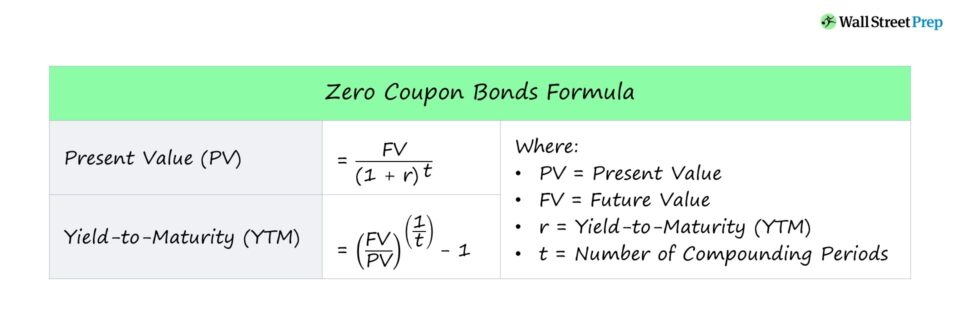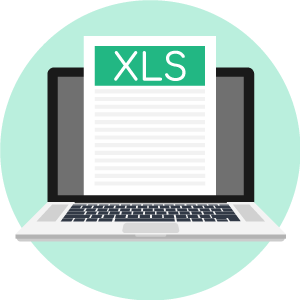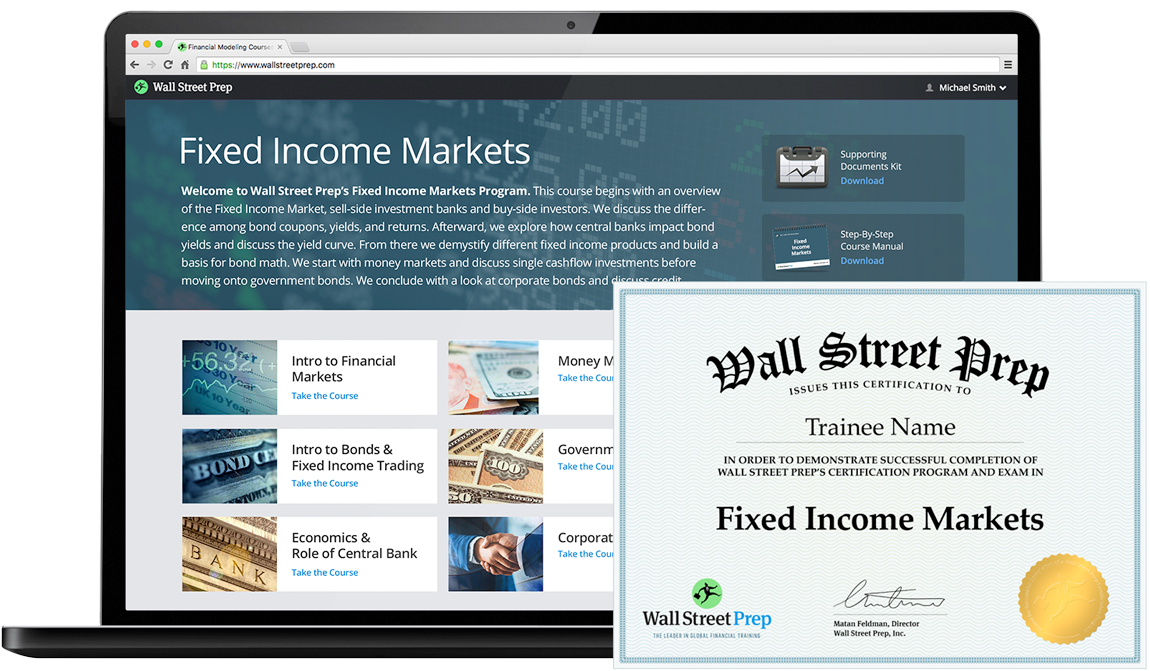# Zero-Coupon Bond

Understand the Structure of Zero-Coupon Bonds• What is the definition of a zero-coupon bond?
• What are the typical features of zero-coupon bonds?
• How can a zero-coupon bond’s price and yield-to-maturity (YTM) be calculated?
• Which risks tend to disproportionally impact zero-coupon bonds?

## Zero-Coupon Bond Features

Zero-coupon bonds, also known as “discount bonds,” are sold by the issuer at a price lower than the face (par) value that is repaid at maturity.

• If Price = 100 ➝ “Par” (Trading at Par Value)
• If Price < 100 ➝ “Discount” (Trading Below Par)

Zero-coupon bonds are debt obligations structured without any required interest payments (i.e. “coupons”) during the lending period, as implied by the name.

Instead, the difference between the face value and price of the bond could be thought of as the interest earned.

Once a zero-coupon bond matures and “comes due,” the investor receives one lump sum payment inclusive of:

• Original Principal
• Accrued Interest
###### Bond Quotes

A bond quote is the current price at which a bond is trading, expressed as a percentage of the par value.

For example, a bond priced at \$900 with a par value of \$1,000 is trading at 90% of its face value, which would be quoted as “90”.

## Zero-Coupon vs Traditional Coupon Bonds

Unlike zero-coupon bonds, traditional coupon bonds with regular interest payments come with the following benefits:

• Source of Recurring Income for Bondholder
• Interest Payments Derisk the Lending (i.e. Raises “Floor” on Maximum Potential Loss)
• Consistent, Timely Interest Payments Confirms Credit Health

In contrast, for zero-coupon bonds, the difference between the face value and the bond’s purchase price represents the bondholder’s return.

Due to the absence of coupon payments, zero-coupon bonds are purchased at steep discounts from their face value, as the next section will explain more in-depth.

## Zero-Coupon Bond – Bondholder Return

The return to the investor of a zero-coupon bond is equal to the difference between the face value of the bond and its purchase price.

In exchange for providing the capital in the first place and agreeing not to be paid interest, the purchase price for a zero-coupon is less than its face value.

The discount on the purchase price is tied to the “time value of money,” as the rate of return must be sufficient to compensate for the potential risk of capital loss.

On the date of maturity – when the zero-coupon bond “comes due” – the bondholder is entitled to receive a lump-sum payment equal to the initial investment amount plus the accrued interest.

Therefore, zero-coupon bonds consist of just two cash flows:

1. Purchase Price: The bond’s market price on the date of purchase (cash inflow to bondholder)
2. Face Value: The bond’s face value repaid in full at maturity (cash outflow to bondholder)

## Zero-Coupon Maturity Length

Generally, zero-coupon bonds have maturities of around 10+ years, which is why a substantial portion of the investor base has longer-term expected holding periods.

Remember, the profit to the investor is not realized until maturity, which is when the bond is redeemed for its full face value, so the length of the holding period must align with the investor’s goals.

### Types of Zero-Coupon Bond Investors

• Pension Funds
• Insurance Companies
• Retirement Planning
• Education Funding (i.e. Long-Term Savings for Children)

Zero-coupon bonds are often perceived as long-term investments, although one of the most common examples is a “T-Bill,” a short-term investment.

U.S. Treasury Bills (or T-Bills) are short-term zero-coupon bonds (< 1 year) issued by the U.S. government.

## Zero-Coupon Bond Price Formula

To calculate the price of a zero-coupon bond – i.e. the present value (PV) – the first step is to find the bond’s future value (FV), which is most often \$1,000.

The next step is to add the yield-to-maturity (YTM) to one and then raise it to the power of the number of compounding periods.

If the zero-coupon bond compounds semi-annually, the number of years until maturity must be multiplied by two to arrive at the total number of compounding periods (t).

### Zero-Coupon Bond Value Formula

• Price of Bond (PV) = FV / (1 + r) ^ t

Where:

• PV = Present Value
• FV = Future Value
• r = Yield-to-Maturity (YTM)
• t = Number of Compounding Periods

## Zero-Coupon Bond Yield-to-Maturity (YTM) Formula

The yield-to-maturity (YTM) is the rate of return received if an investor purchases a bond and proceeds to hold onto it until maturity.

In the context of zero-coupon bonds, the YTM is the discount rate (r) that sets the present value (PV) of the bond’s cash flows equal to the current market price.

To calculate the yield-to-maturity (YTM) on a zero-coupon bond, first divide the face value (FV) of the bond by the present value (PV).

The result is then raised to the power of one divided by the number of compounding periods.

### Zero-Coupon Bond YTM Formula

• Yield-to-Maturity (YTM) = (FV / PV) ^ (1 / t) – 1

## Zero-Coupon Bond Risks

### Interest Rate Sensitivity

One drawback to zero-coupon bonds is their pricing sensitivity based on the prevailing market interest rate conditions.

Bond prices and interest rates have an “inverse” relationship with one another:

• Declining Interest Rates ➝ Higher Bond Prices
• Rising Interest Rates ➝ Lower Bond Prices

The prices on zero-coupon bonds tend to fluctuate based on the current interest rate environment (i.e. they are subject to greater volatility).

For instance, if interest rates rose, then the zero-coupon bond becomes less attractive from a returns perspective.

The bond price must decrease until its yield matches that of comparable debt securities, which reduces the returns to the bondholder.

### “Phantom Income” Taxes

Even though the bondholder technically does not receive interest from the zero-coupon bond, so-called “phantom income” is subject to taxes under the IRS.

However, certain issuances can avoid being taxed, such as zero-coupon municipal bonds and Treasury STRIPS.

## Zero-Coupon Bond Exercise Excel Template

So far, we’ve discussed the features of zero-coupon bonds and how to calculate the bond price and yield-to-maturity (YTM).

We’ll now move to a modeling exercise in Excel, which you can access by filling out the form below.Submitting ...

## Zero-Coupon Bond Price Example Calculation

In our illustrative scenario, let’s say that you’re considering purchasing a zero-coupon bond with the following assumptions.

###### Model Assumptions
• Face Value (FV) = \$1,000
• Number of Years to Maturity = 10 Years
• Compounding Periods (n) = 20 Period (Semi-Annualized)
• Yield-to-Maturity (YTM) = 3.0%

Given those assumptions, the question is, “What price are you willing to pay for the bond?”

If we input the provided figures into the present value (PV) formula, we get the following:

• Present Value (PV) = \$1,000 / (1 + 3.0%) ^ 20
• PV = \$554

The price of this zero-coupon is \$554, which is the estimated maximum amount that you can pay for the bond and still meet your required rate of return.

## Zero-Coupon Bond Yield Example Calculation

In our next section, we’ll work backward to calculate the yield-to-maturity (YTM) using the same assumptions as before.

###### Model Assumptions
• Face Value (FV) = \$1,000
• Number of Years to Maturity = 10 Years
• Compounding Periods (n) = 20 Period (Semi-Annualized)
• Price of Bond (PV) = \$554

We can enter the inputs into the YTM formula since we already have the necessary inputs:

• Yield-to-Maturity (YTM) = (\$1,000 / \$554) ^ (1 / 20) – 1
• YTM = 3.0%

The 3.0% yield-to-maturity (YTM) matches the stated assumption from the earlier section, confirming our formulas are correct.Globally Recognized Certification Program

#### Get the Fixed Income Markets Certification (FIMC©)

Wall Street Prep's globally recognized certification program prepares trainees with the skills they need to succeed as a Fixed Income Trader on either the Buy Side or Sell Side.Inline FeedbacksGet the WSP Fixed Income Markets Certification (FIMC©)

Wall Street Prep’s globally recognized certification program prepares trainees with the skills they need to succeed as a Fixed Income Trader on either the Buy Side or Sell Side.

X

The Wall Street Prep Quicklesson Series

7 Free Financial Modeling Lessons

Get instant access to video lessons taught by experienced investment bankers. Learn financial statement modeling, DCF, M&A, LBO, Comps and Excel shortcuts.